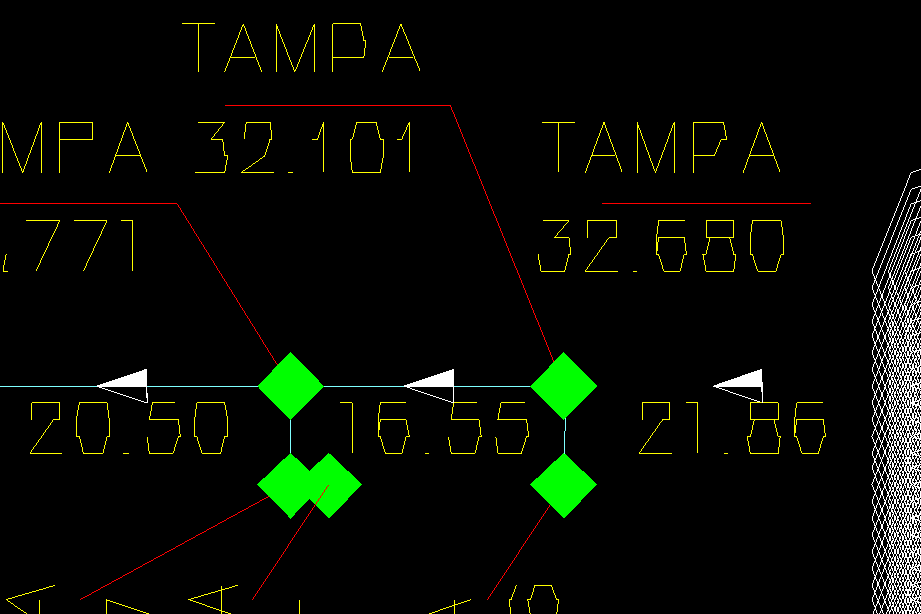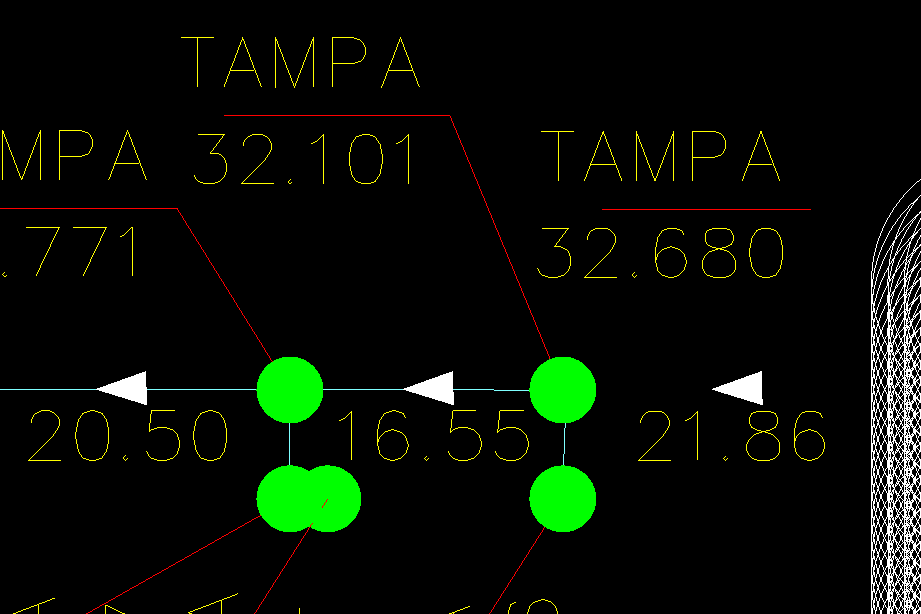# Frequently Asked: How can I solve a large coordinates problem during geometry display and graphics cache auto-regeneration?

## Andrew Markovich

November 22, 2017

Vectorization modules based on DirectX/OpenGL graphics APIs ("WinOpenGL.txv", "WinDirectX.txv" and "WinGLES2.txv") don’t render geometry accurately for large coordinates due to hardware limitations. The GPUs work with 32-bit floats and don’t know anything about 64-bit doubles that represent geometry coordinates, so double->float truncation takes place and causes geometry rendering problems (artifacts) if geometry is far from the origin.

The following example drawing shows double->float truncation artifacts. The geometry looks angular and broken, like it is attracted to a certain grid:Vectorizers can fix this rendering problem, but they require geometry cache regeneration to recompute geometry coordinates into a suitable coordinates system. For example, an application can automatically regenerate geometry cache if an image becomes inaccurate.

This method returns true if the geometry requires regeneration:

``````inline bool requireAutoRegen(OdGsView *pView)
{
OdGsDevice *pDevice = pView->device();
if (!pDevice)
return false;
OdRxDictionaryPtr pProps = pDevice->properties();
if (!pProps.isNull())
{
if (pProps->has(OD_T("RegenCoef")))
{
return OdRxVariantValue(pProps->getAt(OD_T("RegenCoef")))->getDouble() > 1.;
}
}
return false;
}
``````

The RegenCoef vectorization device property represents how many pixels in the current coordinate system will be affected by the double->float conversion. An application can check whether the geometry cache is inaccurate before updating the screen and then execute cache regeneration if this is required:

``````if (requireAutoRegen(pView))
{
m_pDevice->invalidate();
if (m_pDevice->gsModel())
m_pDevice->gsModel()->invalidate(OdGsModel::kInvalidateAll);
}
``````

m_pDevice in this source code example represents the OdGsLayoutHelper class.

Example drawing after geometry regeneration: